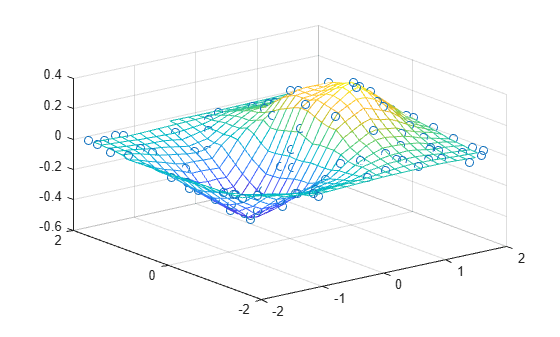Main Content

# TriScatteredInterp

(Not recommended) Interpolate scattered data

`TriScatteredInterp` is not recommended. Use `scatteredInterpolant` instead.

## Description

`TriScatteredInterp` is used to perform interpolation on a scattered dataset that resides in 2-D or 3-D space. A scattered data set defined by locations `X` and corresponding values `V` can be interpolated using a Delaunay triangulation of `X`. This produces a surface of the form `V = F(X)`. The surface can be evaluated at any query location `QX`, using `QV = F(QX)`, where `QX` lies within the convex hull of `X`. The interpolant `F` always goes through the data points specified by the sample.

## Creation

### Syntax

``F = TriScatteredInterp``
``F = TriScatteredInterp(Q,V)``
``F = TriScatteredInterp(X,Y,V)``
``````F = TriScatteredInterp(X,Y,Z,V)``````
``F = TriScatteredInterp(DT,V)``
``F = TriScatteredInterp(___,method)``

### Description

````F = TriScatteredInterp` creates an empty scattered data interpolant.```
````F = TriScatteredInterp(Q,V)` creates an interpolant that fits a surface of the form `V = F(Q)` to the scattered data in (`Q`, `V`). `Q` is a matrix of size `mpts`-by-`ndim`, where `mpts` is the number of points and `ndim` is the dimension of the space where the points reside (`ndim` is 2 or 3). The column vector `V` defines the values at `Q`, where the length of `V` equals `mpts`.```

example

````F = TriScatteredInterp(X,Y,V)` and ```F = TriScatteredInterp(X,Y,Z,V)``` specifies the data point locations in alternative column vector format when working in 2-D and 3-D.```
````F = TriScatteredInterp(DT,V)` uses the specified `DelaunayTri` object `DT` as a basis for computing the interpolant. `DT` is a Delaunay triangulation of the scattered data locations, `DT.X`. The matrix `DT.X` is of size `mpts`-by-`ndim`, where `mpts` is the number of points and `ndim` is the dimension of the space where the points reside, `2 <= ndim <= 3`. `V` is a column vector that defines the values at `DT.X`, where the length of `V` equals `mpts`.```
````F = TriScatteredInterp(___,method)` specifies the interpolation technique `method` used to interpolate the data. You can use any of the previous input argument combinations.```

### Input Arguments

expand all

Scattered data points, specified as a matrix. `Q` is of size `mpts`-by-`ndim`, where `mpts` is the number of points and `ndim` is the dimension of the space where the points reside.

Scattered data points, specified as separate column vectors. Specify (`X`, `Y`) for 2-D data or (`X`, `Y`, `Z`) for 3-D data.

Sample point values, specified as a column vector. `V` defines the values at the sample data points, where the length of `V` equals `mpts`.

Delaunay triangulation representation, specified as a `DelaunayTri` object.

Interpolation method, specified as one of these values:

ValueDescription
`linear`Linear interpolation (default)
`natural`Natural neighbor interpolation
`nearest`Nearest neighbor interpolation

## Properties

expand all

Scattered data points, specified as a matrix. The dimension of `X` is `mpts`-by-`ndim`, where `mpts` is the number of data points and `ndim` is the dimension of the space where the points reside (`2 <= ndim <= 3`).

If column vectors of `X`, `Y` or `X`,`Y`,`Z` coordinates are used to construct the interpolant, then the data is consolidated into a single matrix `X`.

Sample point values, specified as a column vector. `V` is a vector of length `mpts`, where `mpts` is the number of scattered data points.

Interpolation method, specified as one of these values:

ValueDescription
`linear`Linear interpolation (default)
`natural`Natural neighbor interpolation
`nearest`Nearest neighbor interpolation

## Object Functions

To evaluate the interpolant, express the statement in Monge's form ```Vq = F(Xq)```, `Vq = F(Xq,Yq)`, or `Vq = F(Xq,Yq,Zq)` where `Vq` is the value of the interpolant at the query location and `Xq`, `Yq`, and `Zq` are the vectors of point locations.

## Examples

collapse all

Create a data set of 2-D random, scattered points.

```rng default x = rand(100,1)*4-2; y = rand(100,1)*4-2; z = x.*exp(-x.^2-y.^2);```

Construct an interpolant for the data.

`F = TriScatteredInterp(x,y,z);`

Evaluate the interpolant at query point locations `(qx,qy)`. The corresponding interpolated values at these locations are returned in `qz`.

```ti = -2:.15:2; [qx,qy] = meshgrid(ti,ti); qz = F(qx,qy);```

Plot the data and interpolated surface.

```mesh(qx,qy,qz) hold on plot3(x,y,z,'o')```expand all

## See Also

Introduced in R2009a

Download ebook